# Sasa-Satsuma Solitons

## Sasa-Satsuma-mKdV Equation ut + 12 |u|(|u|u)x + uxxx = 0

There are two equivalent forms for the Sasa-Satsuma-mKdV equation:
(1) In complex-variable notation, u = u1 + i u2 satisfies

ut + 6(uux + 3 uxu)u + uxxx =0

(2) In vector notation, u = (u1, u2) satisfies

ut + 12(uu ux + (uux)u) + uxxx =0

### Travelling Wave Solution

Travelling waves are given by the special soliton solution

u(t,x)= (√c /2) exp(iφ)/cosh(√c ξ)

where c > 0 is the speed, −π ≤ φ ≤ π is the phase, and ξ = xct is a moving coordinate.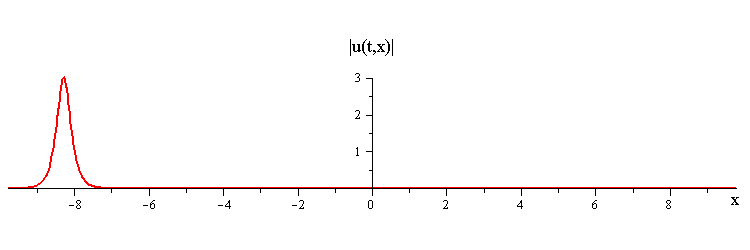### Colliding Travelling-Waves Solution

Collisions where a fast travelling wave with speed c1 and phase φ1 overtakes a slow travelling wave with speed c2 and phase φ2 are given by the special 2-soliton solution

u(t,x)= (c1c2)2 (√σ/2) (c1 exp(iφ1) ( exp(iν1 + √c2 ξ2) + exp(−√c2 ξ2) ) + √c2 exp(iφ2) ( exp(iν2 − √c1 ξ1) + exp(√c1 ξ1) ) )/( 4 √c1c2 cos(φ1 − φ2) + (√c1 − √c2)2 cosh(√c1 ξ1 + √c2 ξ2) + (√c1 + √c2)2σ cosh(√c1 ξ1 − √c2 ξ2) )

with
σ = √( c1 + c2 +2 √c1c2 cos(2(φ1 − φ2)) )/(c1 + √c2 )
and
ν1 = arctan(c2 sin(2(φ1 − φ2)) )/(c1 + √c2 cos(2(φ1 − φ2)) )
ν2 = arctan(c1 sin(2(φ2 − φ1)) )/(c2 + √c1 cos(2(φ2 − φ1)) )

where ξ1 = xc1t, ξ2 = xc2t are moving coordinates.

### Overlay of Travelling-Wave Solutions and Corresponding Collision Solution

Sasa-Satsuma merge-split interaction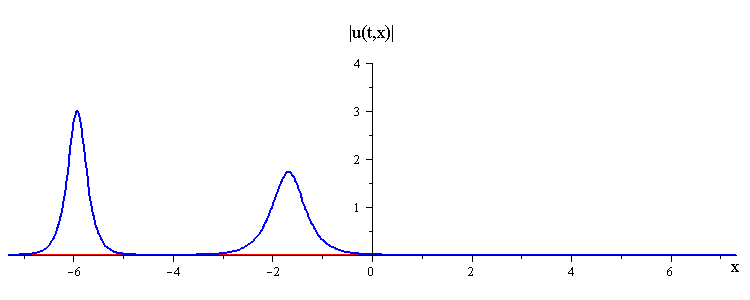ratio of fast to slow wave speeds is c1/c2 = 3
difference in phases is |φ1 − φ2| = 0.6 π

Sasa-Satsuma bounce-exchange interaction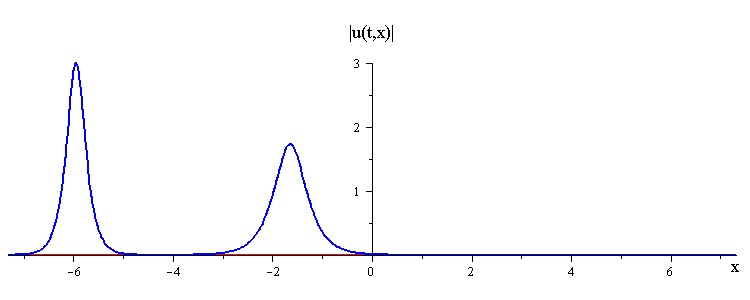ratio of fast to slow wave speeds is c1/c2 = 3
difference in phases is |φ1 − φ2| = 0.35 π

Sasa-Satsuma critical-phase interaction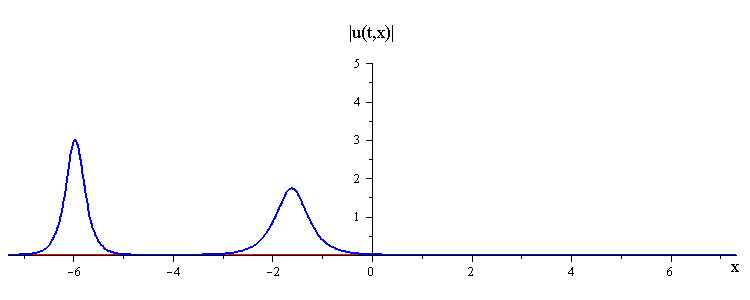ratio of fast to slow wave speeds is c1/c2 = 3
difference in phases is |φ1 − φ2| = 0.70316 π

Sasa-Satsuma absorb-emit interactionratio of fast to slow wave speeds is c1/c2 = 3
difference in phases is |φ1 − φ2| = 0.85 π

Sasa-Satsuma merge-split interaction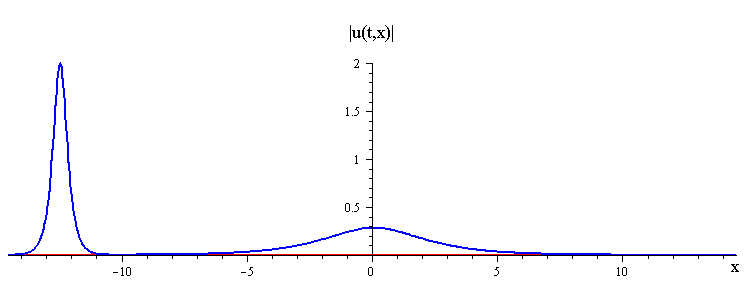ratio of fast to slow wave speeds is c1/c2 = 50
difference in phases is |φ1 − φ2| = 0.35 π
Sasa-Satsuma critical-phase interaction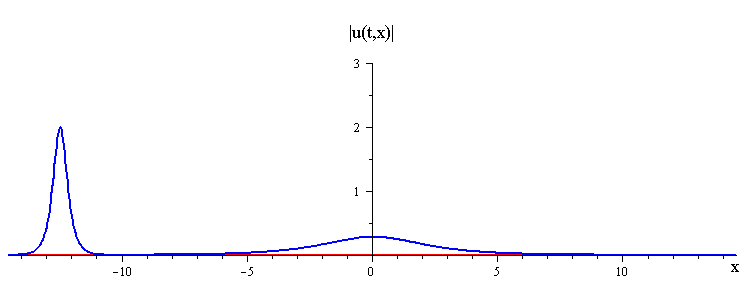ratio of fast to slow wave speeds is c1/c2 = 50
Sasa-Satsuma absorb-emit interaction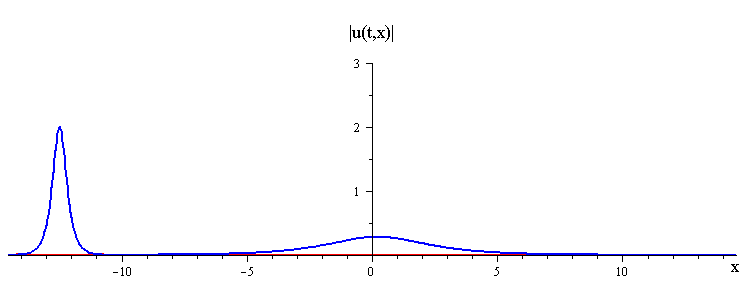ratio of fast to slow wave speeds is c1/c2 = 50